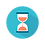From: Bayesian Models for Astrophysical Data, Cambridge Univ. Press

(c) 2017,  Joseph M. Hilbe, Rafael S. de Souza and Emille E. O. Ishida

you are kindly asked to include the complete citation if you used this material in a publication

Code 10.20 Lognormal–logit hurdle model, in R using JAGS, for assessing the relationship between dark-halo mass and stellar mass

==================================================================================

require(R2jags)

# Data

hurdle <- data.frame(x =log(dataB\$Mdm,10), y = asinh(1e10*dataB\$Mstar))

# prepare data for JAGS
Xc <- model.matrix(~ 1 + x,data = hurdle)

Xb <- model.matrix(~ 1 + x, data = hurdle)
Kc <- ncol(Xc)
Kb <- ncol(Xb)

JAGS.data <- list(
Y = hurdle\$y,                                         # response
Xc = Xc,                                                # covariates
Xb = Xb,                                               # covariates
Kc = Kc,                                               # number of betas
Kb = Kb,                                               # number of gammas
N = nrow(hurdle),                                # sample size
Zeros = rep(0, nrow(hurdle)))

# Fit

sink("ZAPGLM.txt")

cat("
model{
# 1A. Priors beta and gamma
for (i in 1:Kc) {beta[i] ~ dnorm(0, 0.0001)}
for (i in 1:Kb) {gamma[i] ~ dnorm(0, 0.0001)}

# 1C. Prior for r parameter
sigmaLN ~ dgamma(1e-3, 1e-3)

# 2. Likelihood (zero trick)
C <- 1e10
for (i in 1:N) {
Zeros[i] ~ dpois(-ll[i] + C)
ln1[i] <- -(log(Y[i]) +log(sigmaLN)+log(sqrt(2*sigmaLN)))
ln2[i] <- -0.5*pow((log(Y[i])-mu[i]),2)/(sigmaLN*sigmaLN)
LN[i] <- ln1[i]+ln2[i]
z[i] <- step(Y[i] - 1e-5)
l1[i] <- (1 - z[i]) * log(1 - Pi[i])
l2[i] <- z[i] * ( log(Pi[i]) + LN[i])
ll[i] <- l1[i] + l2[i]
mu[i] <- inprod(beta[], Xc[i,])
logit(Pi[i]) <- inprod(gamma[], Xb[i,])
}
}"
, fill = TRUE)

sink()

# Define initial values
inits <- function () {
list(beta = rnorm(Kc, 0, 0.1),
gamma = rnorm(Kb, 0, 0.1),
sigmaLN = runif(1, 0, 10) )}

# Identify parameters
params <- c("beta", "gamma", " sigmaLN")

# Run MCMC
H1 <- jags(data = JAGS.data,
inits = inits,
parameters = params,
model = "ZAPGLM.txt",
n.thin = 1,
n.chains = 3,
n.burnin = 5000,
n.iter = 15000)

# Output
print(H1,intervals=c(0.025, 0.975), digits=3)

==================================================================================Output on screen:

Inference for Bugs model at "ZAPGLM.txt", fit using jags,

3 chains, each with 15000 iterations (first 5000 discarded)

n.sims = 30000 iterations saved

mu.vect   sd.vect              2.5%               97.5%     Rhat       n.eff

beta         -2.0990e+00     0.448   -3.1540e+00     -1.1720e+00    1.143         34

beta           5.7800e-01     0.064     4.4500e-01        7.2900e-01    1.114         44

gamma    -5.4561e+01     3.965   -6.3865e+01      -4.8231e+01   1.331         11

gamma     8.0290e+00     0.594    7.0810e+00       9.4220e+00    1.314         11

sigmaLN       2.2100e-01     0.012      1.9900e-01       2.4500e-01     1.003     1100

deviance       3.3600e+13     3.165     3.3600e+13      3.3600e+13     1.000           1

For each parameter, n.eff is a crude measure of effective sample size,

and Rhat is the potential scale reduction factor (at convergence, Rhat=1).

DIC info (using the rule, pD = var(deviance)/2)

pD = 4.9 and DIC = 3.36e+13

DIC is an estimate of expected predictive error (lower deviance is better).